# Principle

The layout of the forest plot is determined by the dataset provided. All the elements of the forestplot are placed in the cells. This makes it very easy to edit any elements in the plot given the row and columns. The graphical parameters of the element can further be editted in the particular cell. Here, we will start with a simple meta analysis forest plot.

The plotting steps demonstrate in this vignette may not necessarily be the optimal. There are other R packages out there are better at the plot demonstrate here. Please choose the best one that suits you. Our final plot is shown in the below.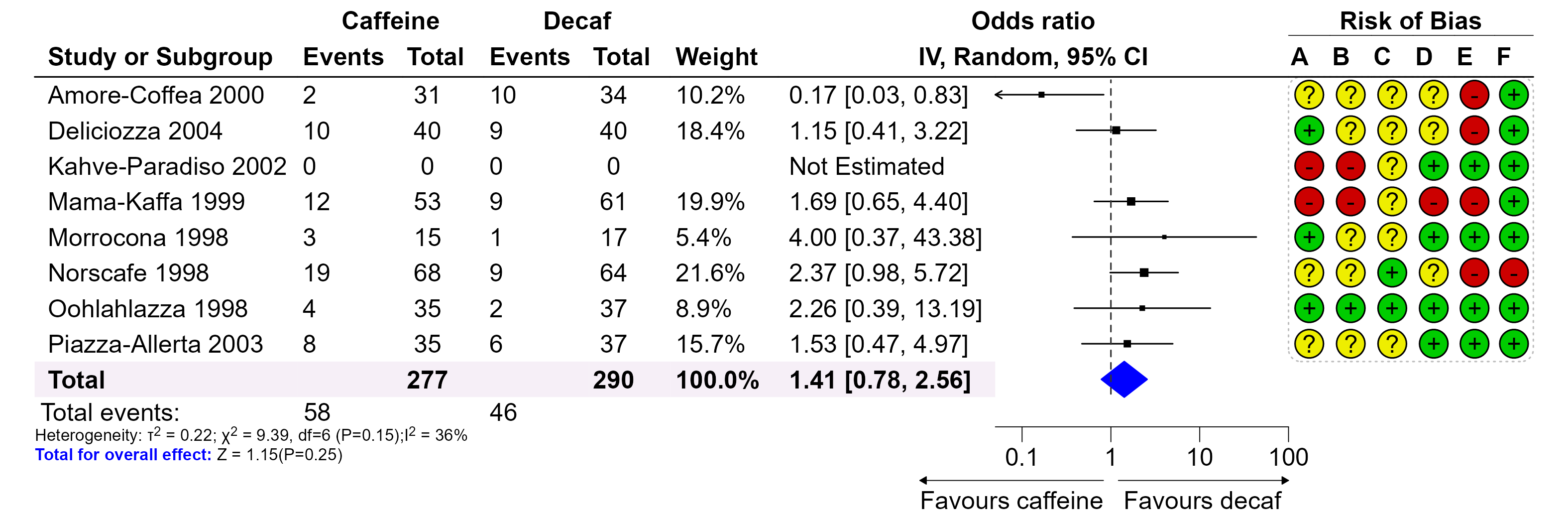# Draw a forest plot

We will start drawing forestplot with an example from metafor, which is similar to the plot above. We first load the data and draw a simple forestplot.

``````library(grid)
library(forestploter)

# Read meta analysis example data

str(dt)
#> 'data.frame':    9 obs. of  16 variables:
#>  \$ author : chr  "Amore-Coffea 2000" "Deliciozza 2004" "Kahve-Paradiso 2002" "Mama-Kaffa 1999" ...
#>  \$ ai     : int  2 10 0 12 3 19 4 8 NA
#>  \$ n1i    : int  31 40 0 53 15 68 35 35 277
#>  \$ ci     : int  10 9 0 9 1 9 2 6 NA
#>  \$ n2i    : int  34 40 0 61 17 64 37 37 290
#>  \$ weights: num  10.19 18.37 NA 19.91 5.37 ...
#>  \$ orci   : chr  "0.17 [0.03, 0.83]" "1.15 [0.41, 3.22]" "Not Estimated" "1.69 [0.65, 4.40]" ...
#>  \$ rb.a   : chr  "?" "+" "-" "-" ...
#>  \$ rb.b   : chr  "?" "?" "-" "-" ...
#>  \$ rb.c   : chr  "?" "?" "?" "?" ...
#>  \$ rb.d   : chr  "?" "?" "+" "-" ...
#>  \$ rb.e   : chr  "-" "-" "+" "-" ...
#>  \$ rb.f   : chr  "+" "+" "+" "+" ...
#>  \$ est    : num  0.166 1.148 NA 1.691 4 ...
#>  \$ lb     : num  0.033 0.409 NA 0.65 0.369 ...
#>  \$ ub     : num  0.829 3.219 NA 4.4 43.383 ...

# Prepare a blank column for the CI
dt\$cicol <- paste(rep(" ", 20), collapse = " ")

# Select some columns for plotting, this will be used as a skeleton of the forestplot
dt_fig <- dt[,c(1:7, 17, 8:13)]

colnames(dt_fig) <- c("Study or Subgroup",
"Events","Total","Events","Total",
"Weight",
"", "",
LETTERS[1:6])

dt_fig\$Weight <- sprintf("%0.1f%%", dt_fig\$Weight)
dt_fig\$Weight[dt_fig\$Weight == "NA%"] <- ""

# Convert NA to blank string
dt_fig[is.na(dt_fig)] <- ""

# Background to white and summary diamond to black
tm <- forest_theme(core = list(bg_params=list(fill = c("white"))),
summary_col = "black",
arrow_label_just = "end",
arrow_type = "closed")

p <- forest(dt_fig,
est = dt\$est,
lower = dt\$lb,
upper = dt\$ub,
sizes = sqrt(dt\$weights/100),
is_summary = c(rep(F, nrow(dt)-1), T),
ci_column = 8,
ref_line = 1,
x_trans = "log",
arrow_lab = c("Favours caffeine","Favours decaf"),
xlim = c(0.05, 100),
ticks_at = c(0.1, 1, 10, 100),
theme = tm)
p``````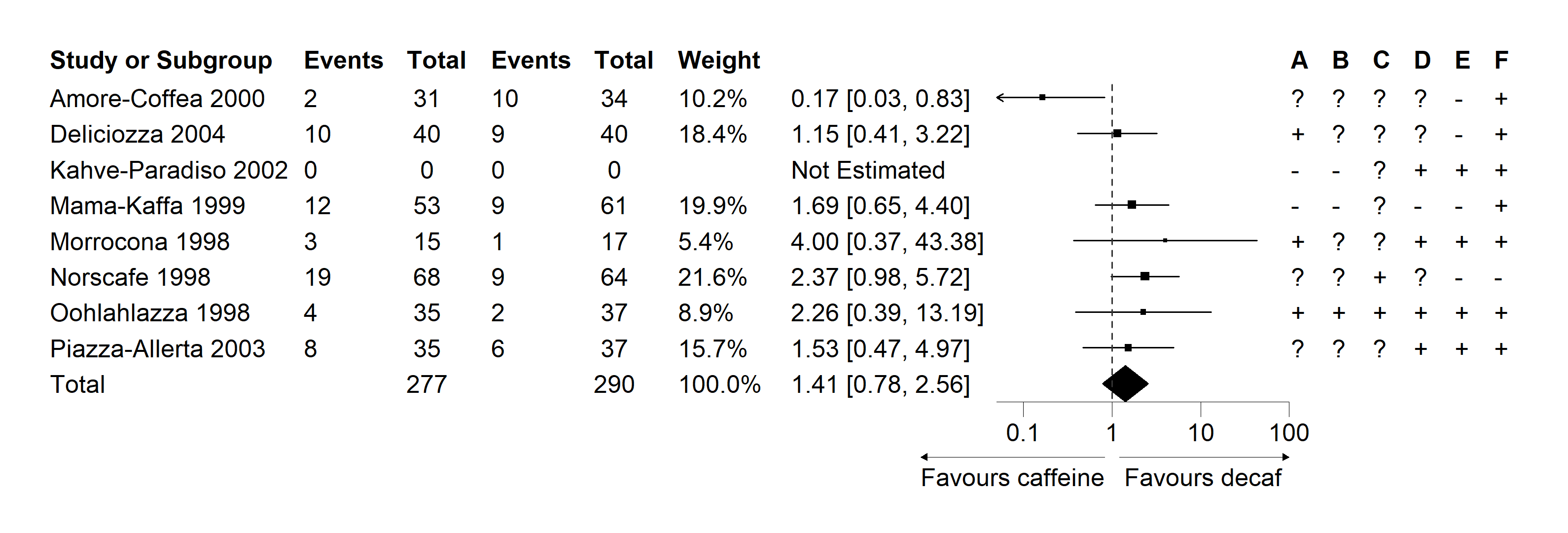# Editing forest plot

The package has some functionality to modify the forestplot. Below is the functions to edit various aspects of the plot:

• The `edit_plot` function can be used to change the graphical parameter of text, background and CI. For example the color or font face of some cells. Other parameters controlling the background and text can be passed, position of the text for example.
• The `add_text` function can be used to add text to certain rows/columns. If you want a complicated text align, you can leave some rows and/or columns blank and use this function to add the text.
• The `insert_text` function can be used to insert a row before or after a certain row and add text. This function can be used to insert text between groups. The idea is to draw all data, and insert text after some rows to separate groups.
• The `add_border` function can be used to add a border to a specific cell.
• The `add_grob` function can be used to add different grobs.

## Edit plot

Below is to make the total row text to bold and change the color of the diamond shape. Then change the background color of the total row. After that, align texts in the last six columns to the center.

``````# Change fontface
g <- edit_plot(p, row = 9,
gp = gpar(fontface = "bold"))

# Change color
g <- edit_plot(g, col = 8, row = 9, which = "ci",
gp = gpar(col = "blue", fill = "blue"))

# Change background of the total row
g <- edit_plot(g, col = 1:7,
row = 9,
which = "background",
gp = gpar(fill = "#f6eff7"))

# Align texts to center
g <- edit_plot(g, col = 9:14,
which = "text",
# gp = gpar(),
hjust = unit(0.5, "npc"),
x = unit(0.5, "npc"))
g``````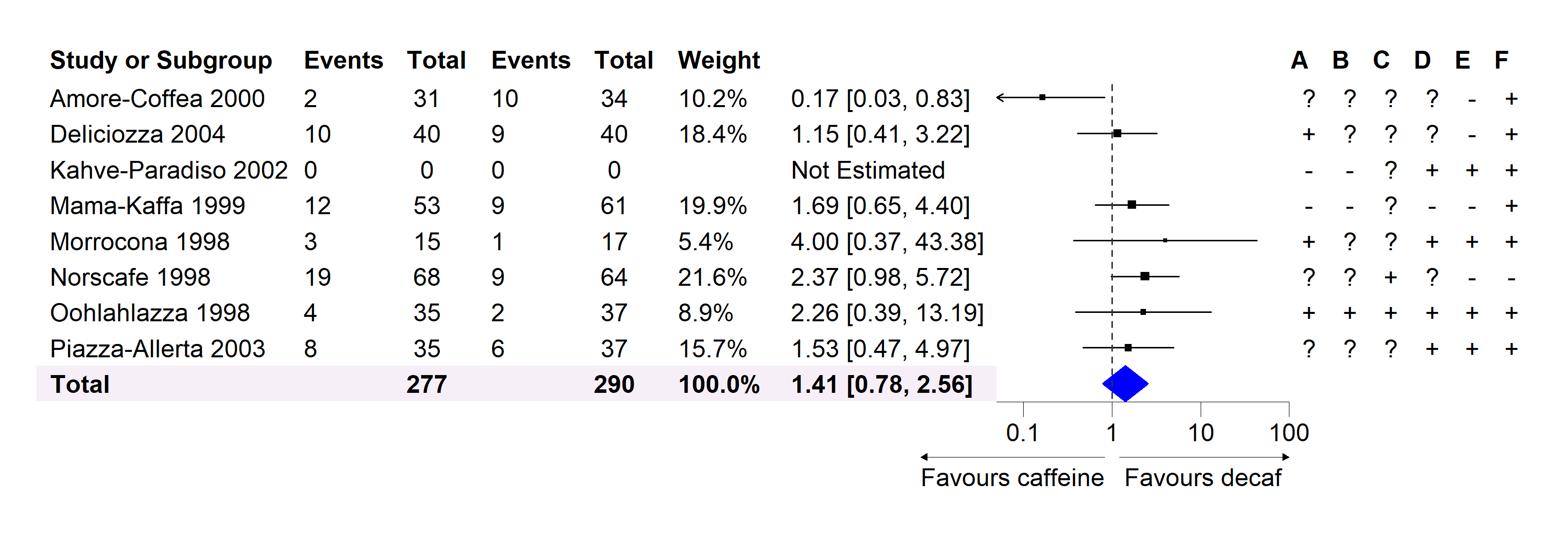For the text align, `hjust = unit(0, "npc")` and `x = unit(0, "npc")` to align left. `hjust = unit(0.5, "npc")` and `x = unit(0.5, "npc")` to align the text in the center. `hjust = unit(1, "npc")` and `x = unit(0.9, "npc")` to align right.

In this step, we want to add some text to the header and total number of events in the data.

``````# Add or insert some text to header on top of CI columns
g <- add_text(g, text = "IV, Random, 95% CI",
col = 7:8,
gp = gpar(fontface = "bold"))

g <- insert_text(g, text = "Odds ratio",
col = 7:8,
gp = gpar(fontface = "bold"))

# Group outcomes
g <- add_text(g, text = "Caffeine",
row = 1,
col = 2:3,
gp = gpar(fontface = "bold"))

g <- add_text(g, text = "Decaf",
row = 1,
col = 4:5,
gp = gpar(fontface = "bold"))

# Add text on the top of risk of bias data
g <- add_text(g, text = "Risk of Bias",
row = 1,
col = 9:14,
gp = gpar(fontface = "bold"))

# Insert event count
g <- insert_text(g,
text = c("Total events:"),
row = 9,
col = 1,
before = FALSE,
just = "left")

# Note: The row counts need to add one to account for
# `insert_text` in the previous step
g <- add_text(g, text = "58",
col = 2,
row = 10,
just = "left")

g <- add_text(g, text = "46",
col = 4,
row = 10,
just = "left")

g``````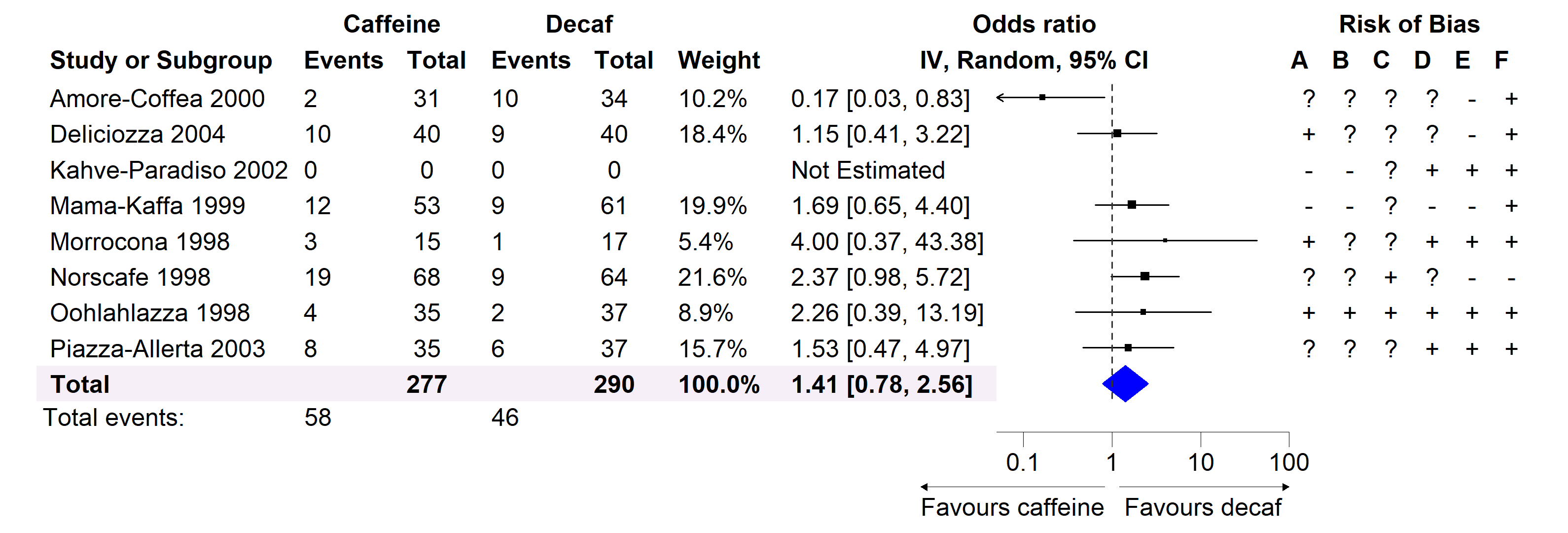The next step we will add some borders at header. The default is to add border at the bottom of the cell(s).

``````# Add or insert some text to header
row = 1,
col = 9:14,
gp = gpar(lwd = .5))

row = 2,
gp = gpar(lwd = 1))

g``````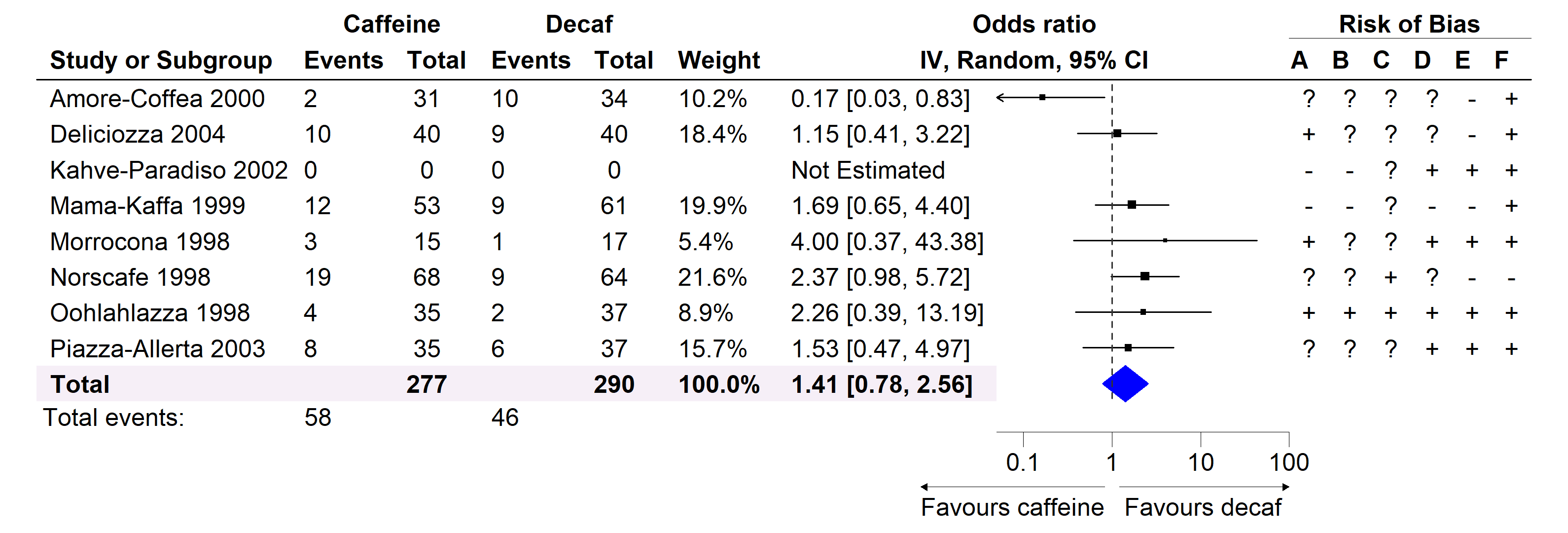In the next step, we will add a rounded rectangle border with dashed line around the risk of bias data. Then draw circle grob with different color at the bottom of the text.

``````g <- add_grob(g,
row = 1:c(nrow(dt_fig) - 1),
col = 9:14,
order = "background",
gb_fn = roundrectGrob,
r = unit(0.05, "snpc"),
gp = gpar(lty = "dotted",
col = "#bdbdbd"))

# Draw circle grob, you can also draw a `pointsGrob`
cols <- c("#eeee00", "#00cc00", "#cc0000")
symb <- c("?", "+", "-")
for(i in seq_along(symb)){
pos <- which(dt_fig == symb[i], arr.ind=TRUE)
for(j in 1:nrow(pos)){
row = pos[j,1],
col = pos[j,2],
order = "background",
gb_fn = circleGrob,
r = 0.4,
gp = gpar(fill = cols[i]))
}
}

g``````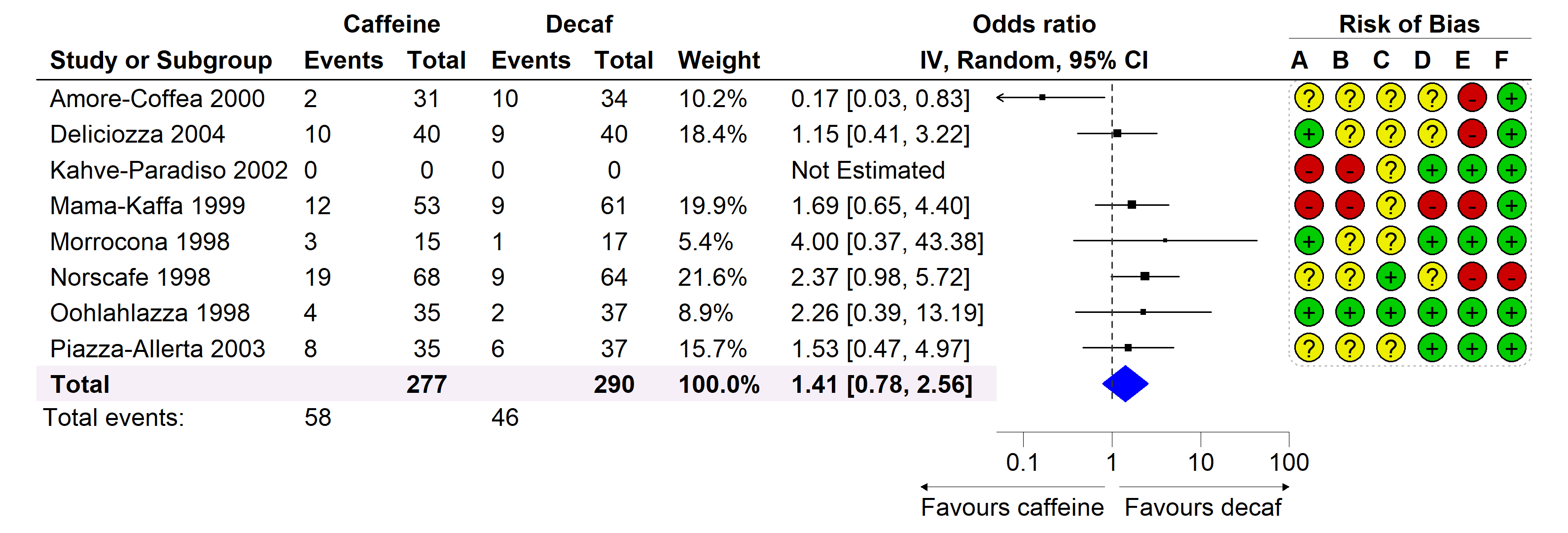The text we are going to create involves with math expression and multiple line. Any one of these can easily be done with `add_text` and setting `parse=TRUE`. We can use the code below to achieve what we want. The line break below is based on the solution here using the `atop` function. You can also use latex2exp package for math expression.

``````txt <- bquote(atop(paste("Heterogeneity: ", tau^2, " = 0.22; ",
chi^2, " = 9.39, df = 6 (P = 0.15)",
I^2, " = 36%"),
"Total for overall effect: Z = 1.15(P=0.25)"))